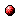DOC HOME SITE MAP MAN PAGES GNU INFO SEARCH

(gmp.info.gz) Floating-point FunctionsInfo Catalog(gmp.info.gz) Rational Number Functions(gmp.info.gz) Top(gmp.info.gz) Low-level Functions

Floating-point Functions
************************

GMP floating point numbers are stored in objects of type `mpf_t' and
functions operating on them have an `mpf_' prefix.

The mantissa of each float has a user-selectable precision, limited
only by available memory.  Each variable has its own precision, and
that can be increased or decreased at any time.

The exponent of each float is a fixed precision, one machine word on
most systems.  In the current implementation the exponent is a count of
limbs, so for example on a 32-bit system this means a range of roughly
2^-68719476768 to 2^68719476736, or on a 64-bit system this will be
greater.  Note however `mpf_get_str' can only return an exponent which
fits an `mp_exp_t' and currently `mpf_set_str' doesn't accept exponents
bigger than a `long'.

Each variable keeps a size for the mantissa data actually in use.
This means that if a float is exactly represented in only a few bits
then only those bits will be used in a calculation, even if the
selected precision is high.

All calculations are performed to the precision of the destination
variable.  Each function is defined to calculate with "infinite
precision" followed by a truncation to the destination precision, but
of course the work done is only what's needed to determine a result
under that definition.

The precision selected for a variable is a minimum value, GMP may
increase it a little to facilitate efficient calculation.  Currently
this means rounding up to a whole limb, and then sometimes having a
further partial limb, depending on the high limb of the mantissa.  But
applications shouldn't be concerned by such details.

The mantissa in stored in binary, as might be imagined from the fact
precisions are expressed in bits.  One consequence of this is that
decimal fractions like 0.1 cannot be represented exactly.  The same is
true of plain IEEE `double' floats.  This makes both highly unsuitable
for calculations involving money or other values that should be exact
decimal fractions.  (Suitably scaled integers, or perhaps rationals,
are better choices.)

`mpf' functions and variables have no special notion of infinity or
not-a-number, and applications must take care not to overflow the
exponent or results will be unpredictable.  This might change in a
future release.

Note that the `mpf' functions are _not_ intended as a smooth
extension to IEEE P754 arithmetic.  In particular results obtained on
one computer often differ from the results on a computer with a
different word size.Info Catalog(gmp.info.gz) Rational Number Functions(gmp.info.gz) Top(gmp.info.gz) Low-level Functions